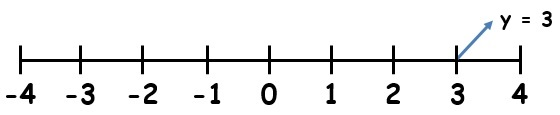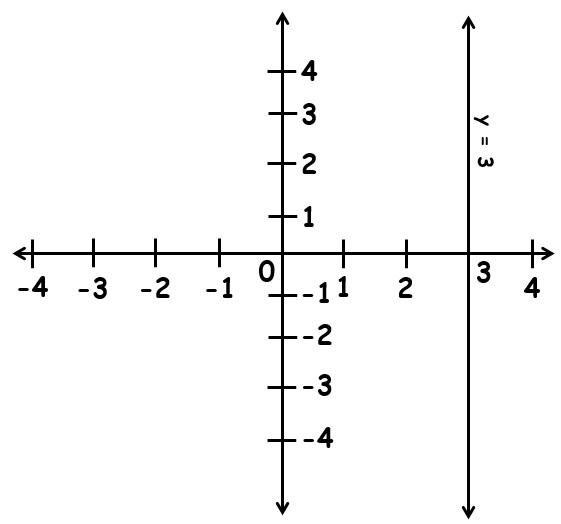# vs.eyeandcontacts.com

## Chapter 4 Linear Equations in Two Variables Exercise 4.4

Question 1: Give the geometric representations of y = 3 as an equation
i) in one variable
ii) in two variables

i)ii)Question 2: Give the geometric representations of 2x + 9 = 0 as an equation
i) in one variable
ii) in two variables